## Electric Dipole Approximation and Selection Rules

We can now expand the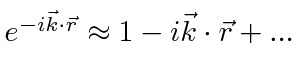term to allow us to compute matrix elements more easily. Sinceand the matrix element is squared, our expansion will be in powers of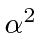which is a small number. The dominant decays will be those from the zeroth order approximation which isThis is called the Electric dipole approximation.

In this Electric Dipole approximation, we can make general progress on computation of the matrix element. If the Hamiltonian is of the formand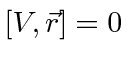, then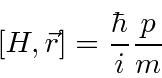and we can writein terms of the commutator.This equation indicates the origin of the name Electric Dipole: the matrix element is of the vector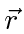which is a dipole.

We can proceed further, with the angular part of the (matrix element) integral.At this point, lets bring all the terms in the formula back together so we know what we are doing.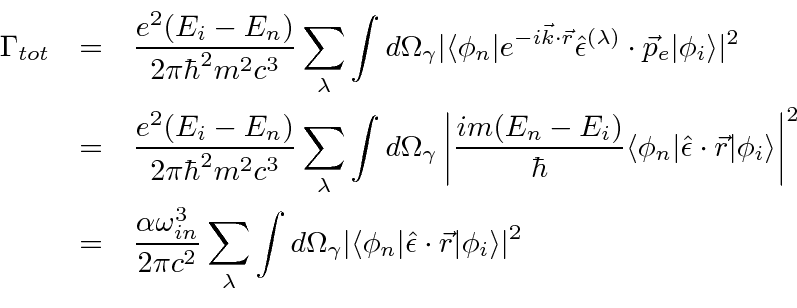This is a useful version of the total decay rate formula to remember.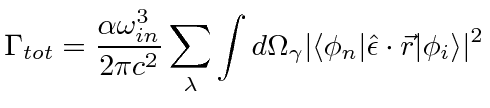We proceed with the calculation to find the E1 selection rules.We will attempt to clearly separate the terms due to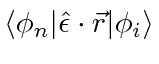for the sake of modularity of the calculation.

The integral with three spherical harmonics in each term looks a bit difficult, but, we can use a Clebsch-Gordan series like the one in addition of angular momentumto help us solve the problem. We will write the product of two spherical harmonics in terms of a sum of spherical harmonics. Its very similar to adding the angular momentum from the twos. Its the same series as we had for addition of angular momentum (up to a constant). (Note that things will be very simple if either the initial or the final state have, a case we will work out below for transitions to s states.) The general formula for rewriting the product of two spherical harmonics (which are functions of the same coordinates) isThe square root and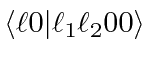can be thought of as a normalization constant in an otherwise normal Clebsch-Gordan series. (Note that the normal addition of the orbital angular momenta of two particles would have product states of two spherical harmonics in different coordinates, the coordinates of particle one and of particle two.) (The derivation of the above equation involves a somewhat detailed study of the properties of rotation matrices and would take us pretty far off the current track (See Merzbacher page 396).)

First add the angular momentum from the initial state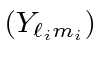and the photonusing the Clebsch-Gordan series, with the usual notation for the Clebsch-Gordan coefficients.I remind you that the Clebsch-Gordan coefficients in these equations are just numbers which are less than one. They can often be shown to be zero if the angular momentum doesn't add up. The equation we derive can be used to give us a great deal of information.We know, from the addition of angular momentum, that adding angular momentum 1 tocan only give answers in the range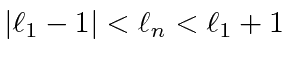so the change in inbetween the initial and final state can only be. For other values, all the Clebsch-Gordan coefficients above will be zero.

We also know that theare odd under parity so the other two spherical harmonics must have opposite parity to each other implying that, thereforeWe also know from the addition of angular momentum that the z components just add like integers, so the three Clebsch-Gordan coefficients allowWe can also easily note that we have no operators which can change the spin here. So certainlyWe actually haven't yet included the interaction between the spin and the field in our calculation, but, it is a small effect compared to the Electric Dipole term.

The above selection rules apply only for the Electric Dipole (E1) approximation. Higher order terms in the expansion, like the Electric Quadrupole (E2) or the Magnetic Dipole (M1), allow other decays but the rates are down by a factor ofor more. There is one absolute selection rule coming from angular momentum conservation, since the photon is spin 1. No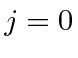totransitions in any order of approximation.

As a summary of our calculations in the Electric Dipole approximation, lets write out the decay rate formula.

Jim Branson 2013-04-22